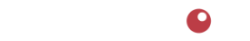# Price Increase Calculator

This calculator determines the price of a product that is periodically sold at a discount to ensure the same overall profit margin will be earned as if the product were not sold at a discount.

The base price is the product price when no discounts are applied.
Total number of periods is usually 52 (weeks) or 12 (months).
Number of discount periods is the number of periods the product will be sold at a discounted price. Discount is the % discount entered as decimal numbers - for example, 15% would be 0.15, 25% would be 0.25, etc.

To use this calculator, enter the base price of the product, total number of periods, number of periods the product price will be discounted, and the discount expressed as a decimal number. Then press the [Calculate] button.
The calculated increased price will appear in the space labeled "New Price".

Be sure to check out our other calculators located here.

### Price Increase Calculator

Explanation:

Special thanks to Barbara "Alge-Barbara" Kutasz4110 SE Hawthorne Blvd. #739

Portland OR 97214

503.234.7199

Notice | Privacy